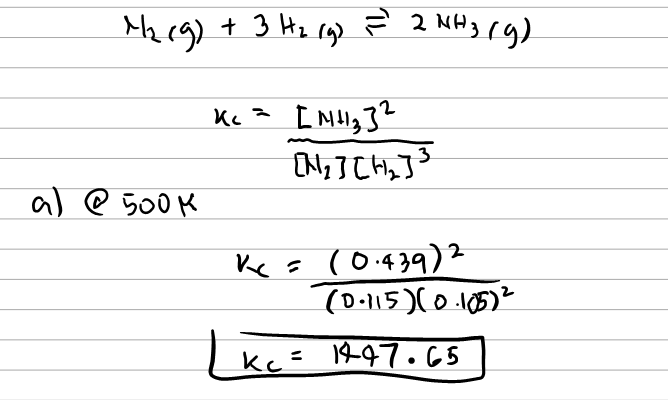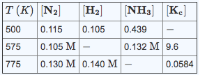# Problem: Consider the following reaction N2 (g) + 3H2 (g) ⇌ 2NH3 (g) Complete the following table. Assume that all concentrations are equilibrium concentrations in M. Part A Find Kc at 500 K. Part B Find [H2] at 575 K. Part C Find [NH3] at 775 K.

###### FREE Expert Solution
95% (192 ratings)
###### FREE Expert Solution95% (192 ratings)###### Problem Details

Consider the following reaction

N2 (g) + 3H2 (g) ⇌ 2NH3 (g)

Complete the following table. Assume that all concentrations are equilibrium concentrations in M.Part A

Find Kc at 500 K.

Part B

Find [H2] at 575 K.

Part C

Find [NH3] at 775 K.

What scientific concept do you need to know in order to solve this problem?

Our tutors have indicated that to solve this problem you will need to apply the Equilibrium Expressions concept. If you need more Equilibrium Expressions practice, you can also practice Equilibrium Expressions practice problems.

What is the difficulty of this problem?

Our tutors rated the difficulty ofConsider the following reaction N2 (g) + 3H2 (g) ⇌ 2NH3 (g) ...as medium difficulty.

How long does this problem take to solve?

Our expert Chemistry tutor, Sabrina took 9 minutes and 44 seconds to solve this problem. You can follow their steps in the video explanation above.

What professor is this problem relevant for?

Based on our data, we think this problem is relevant for Professor DeSimone's class at UA.